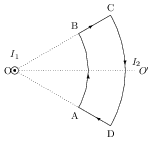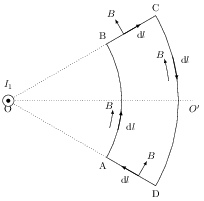# Effect of a Uniform Magnetic Field on a Current Loop

## Problems from IIT JEE

Problem (IIT JEE 2006):An infinitely long wire carrying current $I_1$ passes through O and is perpendicular to the plane of paper. Another current carrying loop ABCD lies in plane of paper as shown in figure. Which of the following statement(s) is (are) correct?

1. net force on the loop is zero.
2. net torque on the loop is zero.
3. loop will rotate clockwise about axis $OO^\prime$ when seen from $O$.
4. loop will rotate anticlockwise $OO^\prime$ when seen from $O$.

Solution:The magnetic field $\vec{B}$ by the current $I_1$ is circumferential. For each element $\mathrm{d}\vec{l}$ on the branch AB, $\vec{B}$ is parallel to the current direction making the force, \begin{alignat}{2} &\vec{F}_\text{AB}=\int_{A}^{B} I_2\,\mathrm{d}\vec{l}\times\vec{B}=0.\nonumber \end{alignat} For the branch CD, $\vec{B}$ is anti-parallel to $\mathrm{d}\vec{l}$. Thus, total force on branch CD is $\vec{F}_\text{CD}=0$.

For the branch BC, $\vec{B}$ is perpendicular to $\mathrm{d}\vec{l}$, and $\mathrm{d}\vec{l}\times \vec{B}$ is coming out of the paper making $\vec{F}_\text{BC}=F_\text{BC}\odot$, where $F_\text{BC}$ is the magnitude of force. For the branch DA, $\mathrm{d}\vec{l}\times \vec{B}$ is going into the paper making $\vec{F}_\text{DA}=F_\text{DA}\otimes$, where $F_\text{DA}$ is the magnitude of force. By symmetry, $\vec{F}_\text{BC}=-\vec{F}_\text{DA}$.

Thus, net force acting on ABCDA is zero. However, there is non-zero clockwise (when looking from O) torque that rotates the loop in clockwise direction. The readers are encouraged to show that, \begin{alignat}{2} &F_\text{BC}=\int_{r_1}^{r_2} I_2 B(r) \mathrm{d} r=\frac{\mu_0 I_1 I_2}{2\pi}\ln\left(\frac{r_2}{r_1}\right). \nonumber \end{alignat} Thus, correct options are A and C.